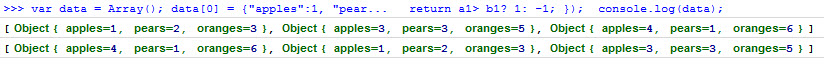# jquery sort array by index

Quick example on how to use JavaScript to sort arrays by index values. To analyse the best method of doing this in terms of performance I looked at a JS perf test for sorting objects.

``````var data = Array();
data = {"apples":1, "pears":2, "oranges":3};
data = {"apples":3, "pears":3, "oranges":5};
data = {"apples":4, "pears":1, "oranges":6};

console.log(data);

data.sort(function(a, b){
var a1= a.pears, b1= b.pears;
if(a1== b1) return 0;
return a1> b1? 1: -1;
});

console.log(data);``````

Here you can see that we have sorted by “pears” values. The first line is before and second line is after the sort: pear 1, pear 2, pear 3.### JS Sort on Objects

``````//objects
var array = [{id:'12', name:'Smith', value:1},{id:'13', name:'Jones', value:2}];
array.sort(function(a, b){
var a1= a.name, b1= b.name;
if(a1== b1) return 0;
return a1> b1? 1: -1;
});``````

### JS Sort on Arrays

``````//arrays
var array =[ ['12', ,'Smith',1],['13', 'Jones',2]];
array.sort(function(a, b){
var a1= a, b1= b;
if(a1== b1) return 0;
return a1> b1? 1: -1;
});``````